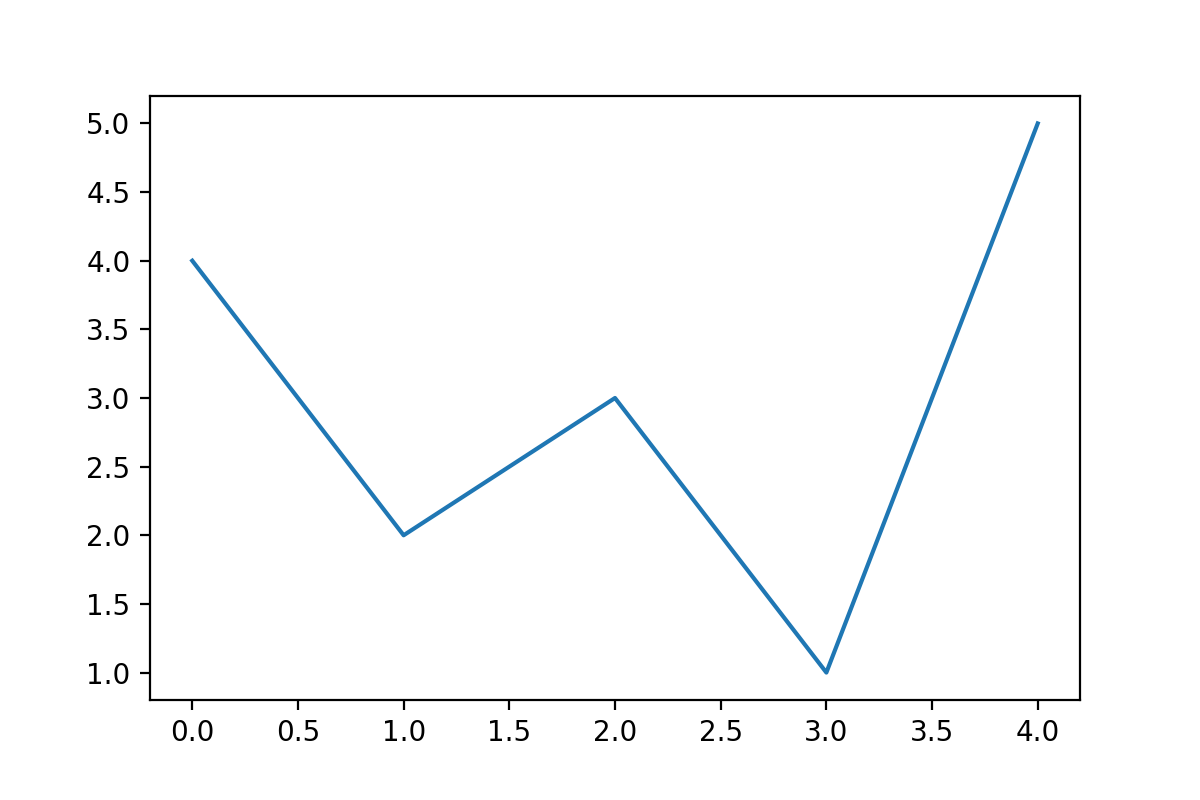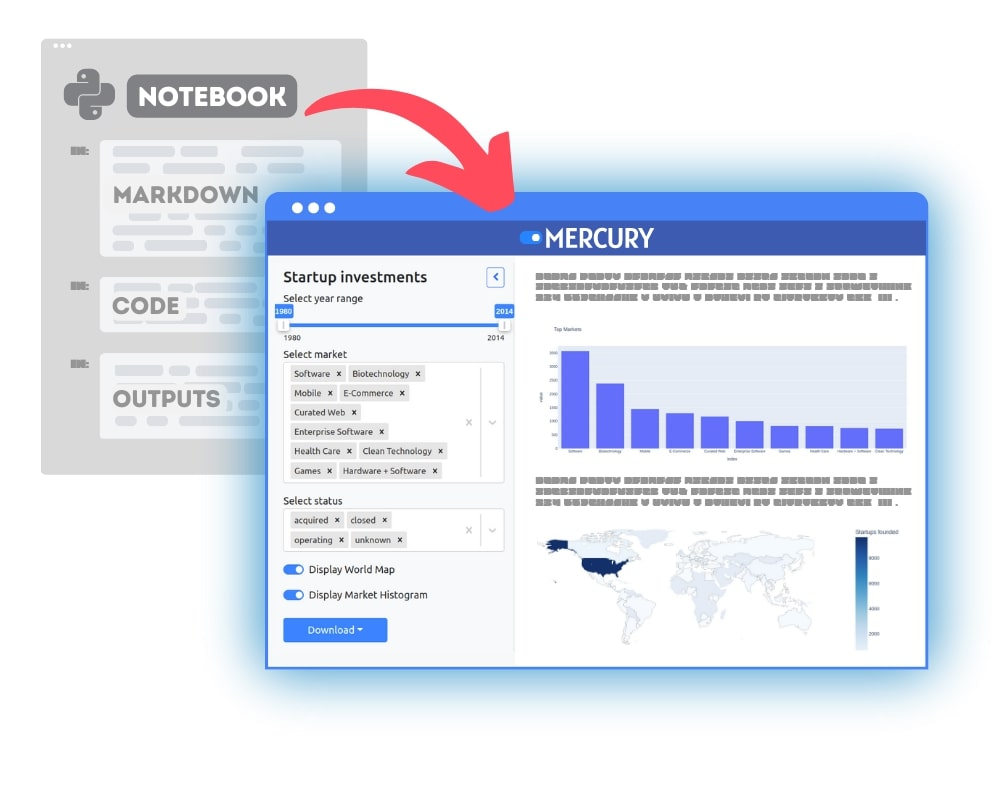The `Matplotlib` is a popular plotting library for Python. It can be used in Python scripts and Jupyter Notebooks. The plot can be displayed in a separate window or a notebook. What if you would like to save the plot to a file? In this article, I will show you how to save the `Matplotlib` plot into a file. It can be done by using 14 different formats.

## Quick code snippet

Below is a quick Python code snippet that shows how to save a figure to a file.

``````import matplotlib.pyplot as plt

# create a plot
plt.plot([4,2,3,1,5])

# save figure
plt.savefig("my_plot.png")
``````

Important:

1. Please call `plt.show()` after `plt.savefig()`. Otherwise, you will get an empty plot in the file.
2. For Jupyter Notebook users, you need to call `plt.savefig()` in the same cell in which the plot is created. Otherwise, the file has an empty figure.

## The `savefig` function - deep dive

All plots created with `Matplotlib` are saved with `savefig` function. Please take a while a look into `savefig` documentation.

Below are `savefig` arguments that you might find useful:

• `fname` - a path where to save a plot. It can be without extension. For `fname` with the extension included the `format` will be automatically selected. Common extensions: `.png`, `.pdf`, `.svg`;
• `dpi` - a numeric value for dots per inch; it controls the size of the figure in pixels. You can read more in an article on how to change figure size in `Matplotlib`;
• `bbox_inches` - controls the white space around the figure, if you don’t need margins, please set this parameter to `tight`;
• `transparent` - is a boolean value that can turn the plot background into transparent;
• `format` - is a string with the output file format. Common formats are `png`, `pdf`, `svg`. If not specified, it is automatically set from the `fname` extension. The default is `png`.

You can list all available formats in `Matplotlib` with below Python code:

``````import matplotlib.pyplot as plt
print(plt.gcf().canvas.get_supported_filetypes())
``````

Output:

``````{
'eps': 'Encapsulated Postscript',
'jpg': 'Joint Photographic Experts Group',
'jpeg': 'Joint Photographic Experts Group',
'pdf': 'Portable Document Format',
'pgf': 'PGF code for LaTeX',
'png': 'Portable Network Graphics',
'ps': 'Postscript',
'raw': 'Raw RGBA bitmap',
'rgba': 'Raw RGBA bitmap',
'svg': 'Scalable Vector Graphics',
'svgz': 'Scalable Vector Graphics',
'tif': 'Tagged Image File Format',
'tiff': 'Tagged Image File Format'
}
``````

There are, in total 13 formats supported by `Matplotlib`.Let’s try to save a figure to PNG format with transparent background with a size of `1200x800` pixels:

``````import matplotlib.pyplot as plt
plt.figure(figsize=(6,4), dpi=200)
plt.plot([4,2,3,1,5])
plt.savefig("my_plot.png", transparent=True)
``````

We set the size of the figure during creation, the size in pixels is computed by multiplying `figsize` by `dpi`.## Save `Matplotlib` figure

Earlier, we save plots in graphics formats. What if we would like to save the whole `Matplotlib` figure for further manipulation? It is possible. We can use the `pickle` library. It is a Python object serialization package. It doesn’t need to be installed additionally.

Here is an example Python code that creates the `Matplitlib` figure, saves to a file, and loads it back:

``````import pickle
import matplotlib.pyplot as plt

# create figure
fig = plt.figure()
plt.plot([4,2,3,1,5])

# save whole figure
pickle.dump(fig, open("figure.pickle", "wb"))

``````

## Summary

You can save the `Matplotlib` plot to a file using 13 graphics formats. The most common formats are `.png`, `.pdf`, `.svg` and `.jpg`. The `savefig()` function has useful arguments: `transparent`, `bbox_inches`, or `format`. There is an option to serialize the figure object to a file and load it back with the `pickle` package. It can be used to save the figure for future manipulations.### Convert Python Notebooks to Web Apps

We are working on open-source framework Mercury for converting Jupyter Notebooks to interactive Web Applications.### Articles you might find interesing